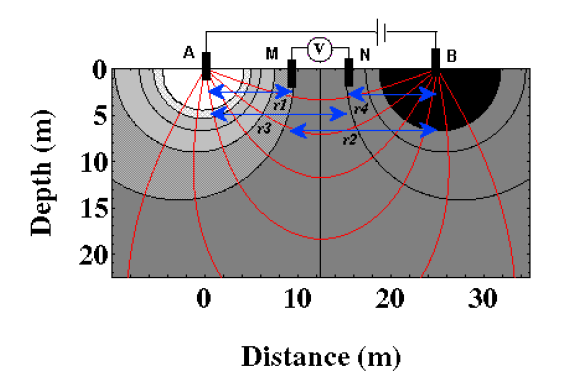Landviser, LLC Enlightening Research
 Search this site: this site has not been updated since 2017... visit us @ landviser.com  to learn about new products and services!

# Electrical profiling

The uniform static electrical field can be created in field conditions to measure soil electrical resistivity or conductivity in situ (Petrovsky, 1925). However, most modern geophysical methods, such as four-electrode profiling and vertical electrical sounding apply non-uniform electrical field to soils through the point electrodes (Figure 2). The electrical resistivity measured with these methods is termed apparent or bulk electrical resistivity, to distinguish it from the resistivity measured in laboratory in homogeneous samples with uniform electrical field. The electrical profiling method is based on the same four-electrode principle as the conductivity cell (Figure 2). The electrical field is distributed in a soil volume, which size can be estimated from the distance among AMNB electrodes. The geometric factor (K) can be precisely derived from the array geometry based on the law of electrical field distribution. Using the Laplace's equation in polar coordinates, Keller and Frischknecht (1966) derived the electrical potential functions around the source (A and B) and measuring (M and N) electrodes. The geometric factor K can be obtained for central-symmetric four-electrode array of AMNB configuration (Figure 2) aswhere [AM], [AN], and [MN] are the distances (m) between the respective electrodes.Figure 2 Scheme of the four-electrode method. Electrical field lines are shown with thin curvilinear lines (non-uniform electrical field).

The arrays of different geometries are suitable for various applications. Equally spaced arrays (AM=MN=NB=a) in the Wenner configuration with small a distances from 2 to 6 cm were used for measurement of electrical resistivity on the walls of open soil pits. Arrays with a from 15 to 80 cm were applied for mapping of lateral changes in electrical resistivity on the soil surface. The electrode array is moved along a surveyed line and the electrical measurements result in a horizontal profile of apparent resistivity. The final results include subsurface apparent resistivity values from the measured locations. Results may be plotted as profile lines or contour maps (isopleth resistivity map), or in other presentations according to the specific needs. The method is more accurate than electromagnetic profiling although slower and more labor-effective.